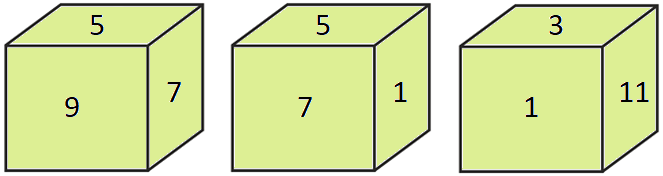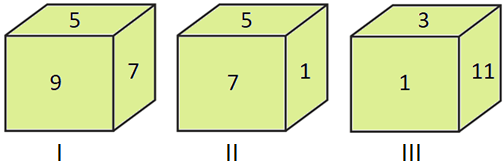# QOTD - Logical Reasoning Question on Cubes and Dices

2019-09-23 | Team PendulumEdu

Three different positions of the same dice are shown below. Which number can be on the face opposite to the face showing ‘3’?Options:

A. 9

B. 5

C. 7

D. 1

Solution:

Consider the given positions of diceTo compare two different positions of dice to find out the numbers opposite to faces on the dice we should follow the following steps:

• First, find the common number/symbol on both the positions of dice
• Then, starting from the common number move in the clockwise direction in both the dice
• While moving in clockwise direction note down the other numbers/symbols
• Lastly, compare the numbers/symbols thus obtained. The next numbers after the common number in both the positions of the dice will be on the opposite faces of the dice.

NOTE: The above logic cannot be applied to the positions of the dice on which more than one face has common number or symbols. For such cases, the non-common numbers will be opposite to each other.

Therefore, analyzing position II and position III of dice, we can say that there is ‘1’ common in both the positions of dice

So,

For position II:

The sequence of the numbers by the above logic will be

1, 7, 5

For position III:

The sequence of the numbers by the above logic will be

1, 3, 11

Now, from the above logic, we can say that ‘7’ will be the number on the face opposite to the face on which ‘3’ is present.

Hence, (C) is the correct answer.

Such type of question is asked in the Logical reasoning section of various exams like SSC CGL, SSC MTS,  SSC CPO, SSC CHSL, RRB JE, RRB NTPC, RRB GROUP D, etc. Try and attempt free mock tests at PendulumEdu and upgrade your preparation.0